# Time machine formula

The movie ``The time machine" IMBD link features some black and green boards with mathematical formulas.

MOV, Ogg Webm. Some of the formulas are a bit strange.
• What does 4'' mean on this frame. It might not be a 4 but a psi.
• On this frame one can see the gradient of a potential phi as well as a magnetic flux and something which looks like the divergence theorem. One can also make up a Maxwell equation for the curl of E.
• There is an elliptic integral on this frame, where lambda is both integrated over as well as is in the lower bound. Tr{ W2 } probably means trace. The 8 π T appears in the Einstein Field equations. The ∇2 A in the wave equation for the electro magnetic potential A.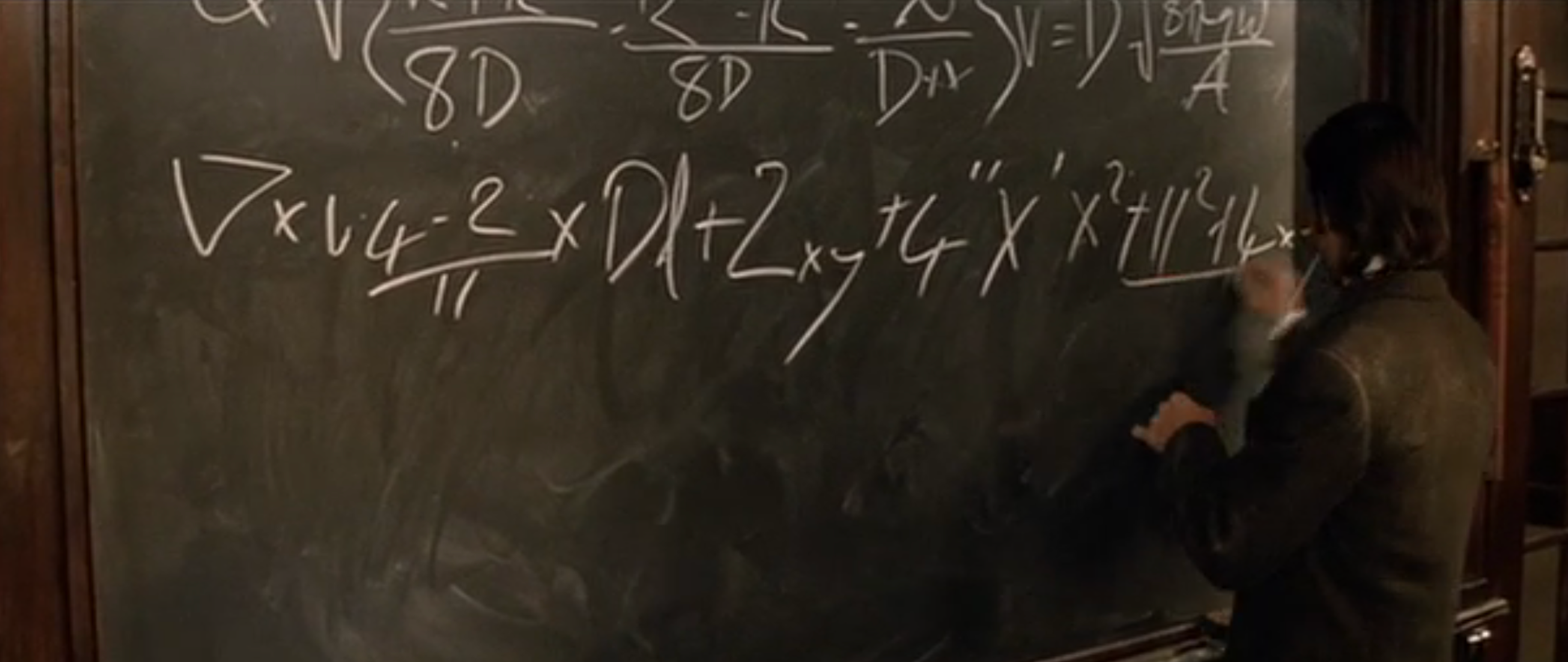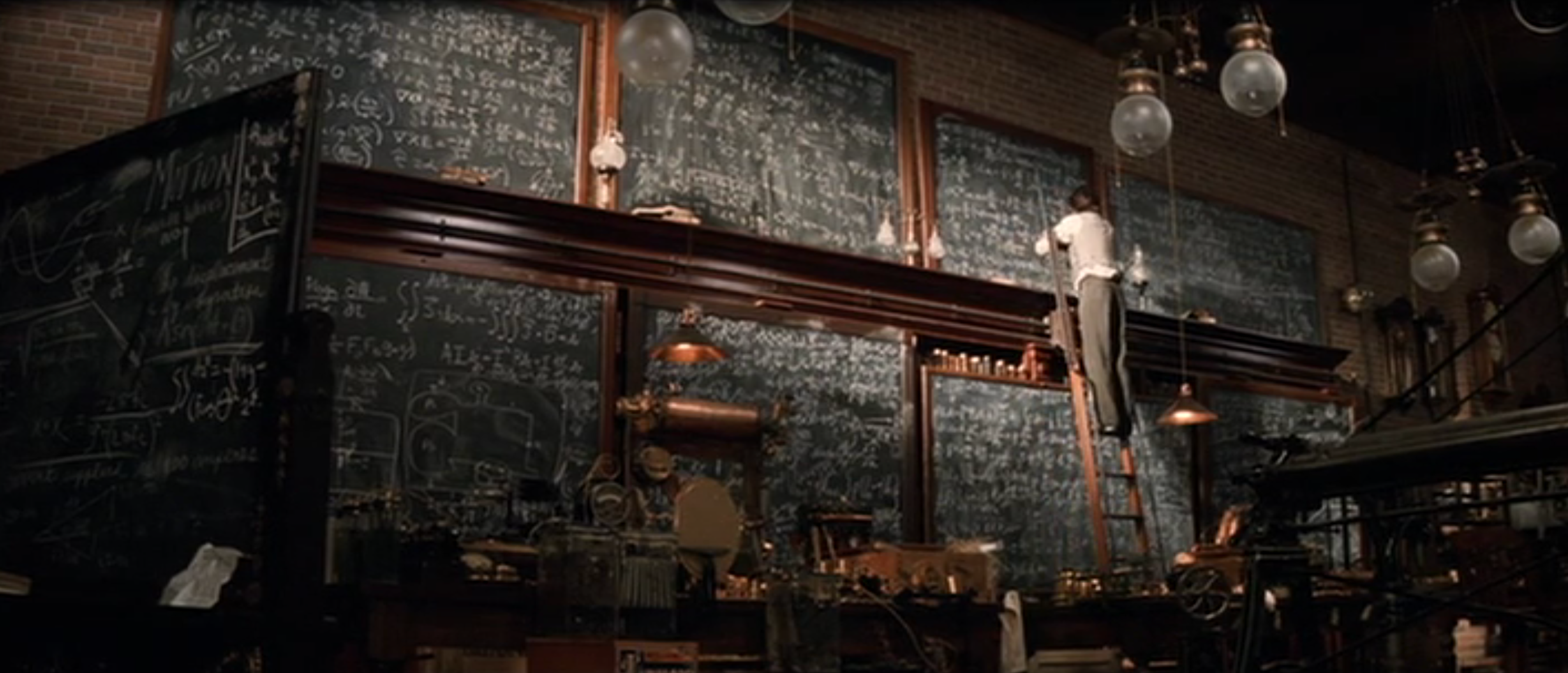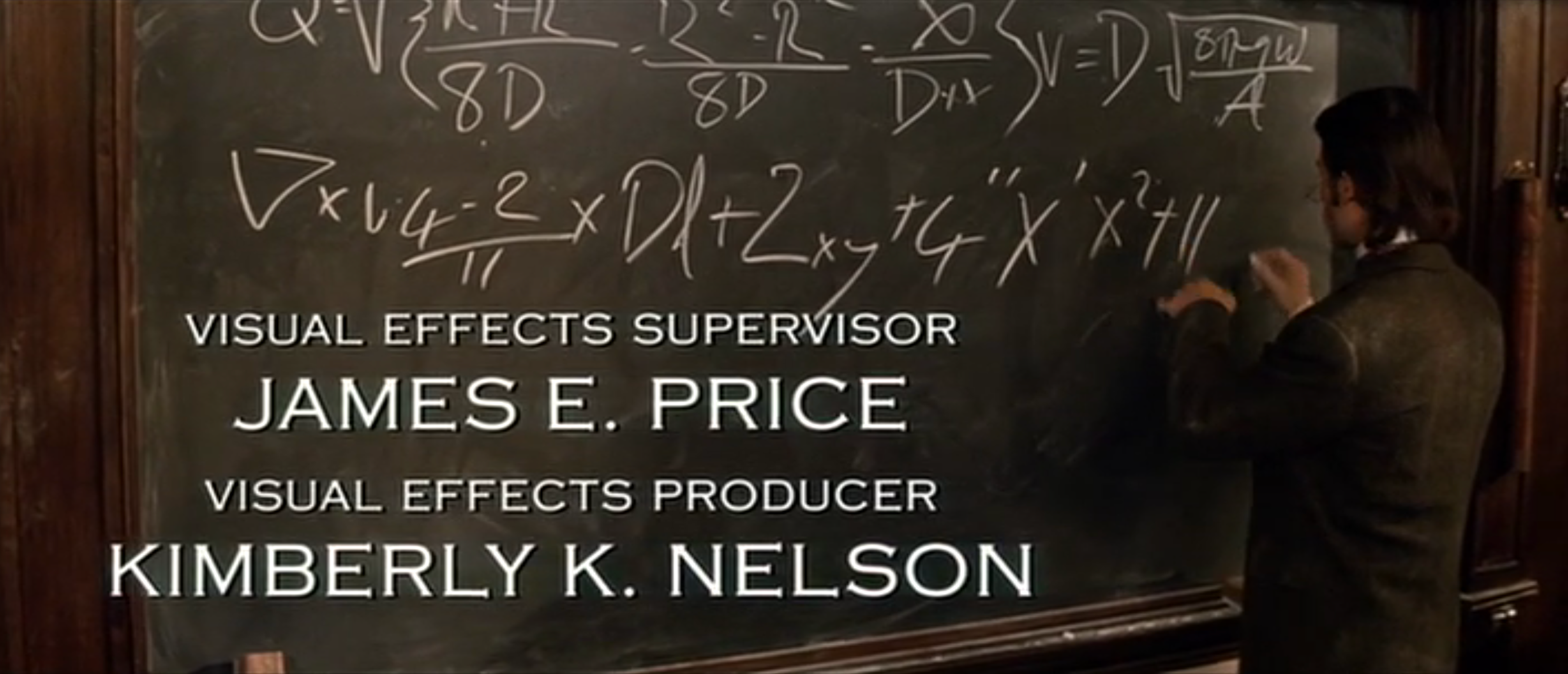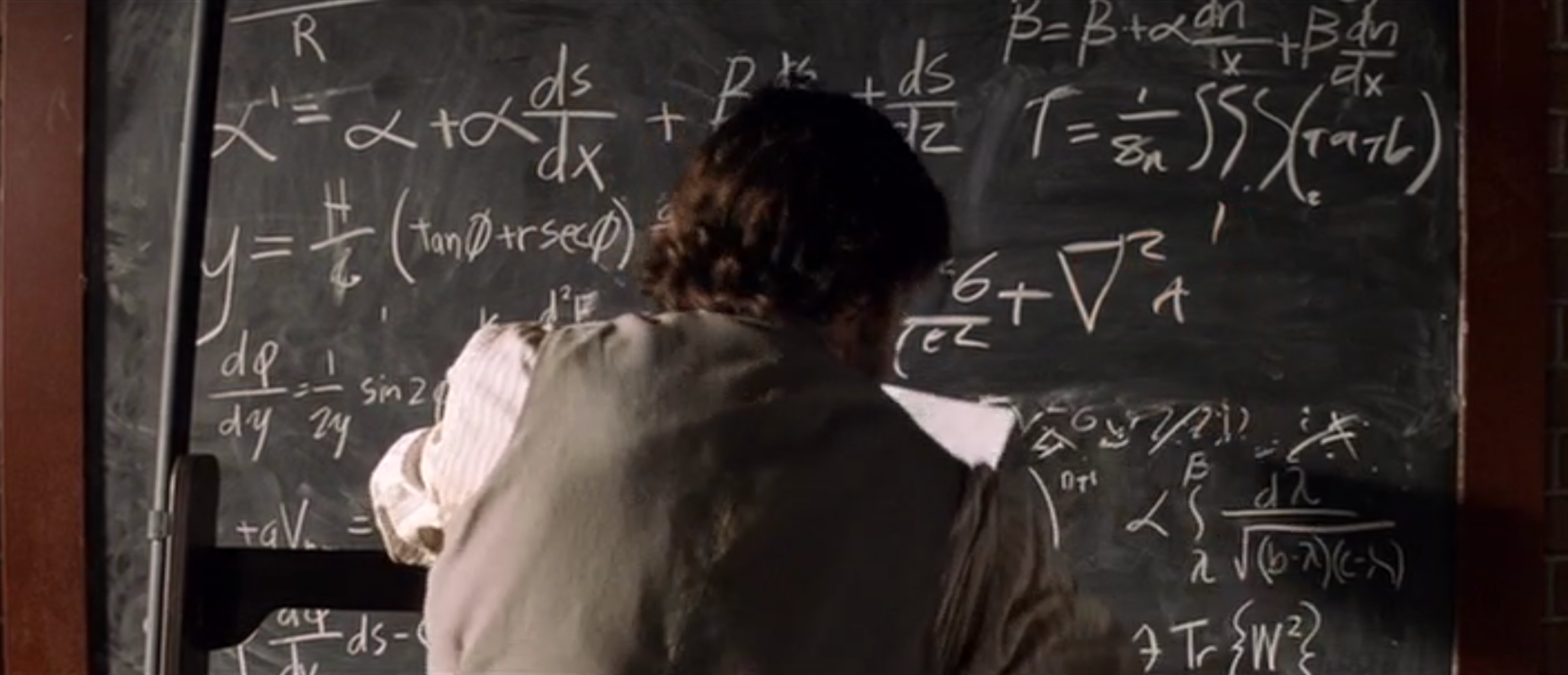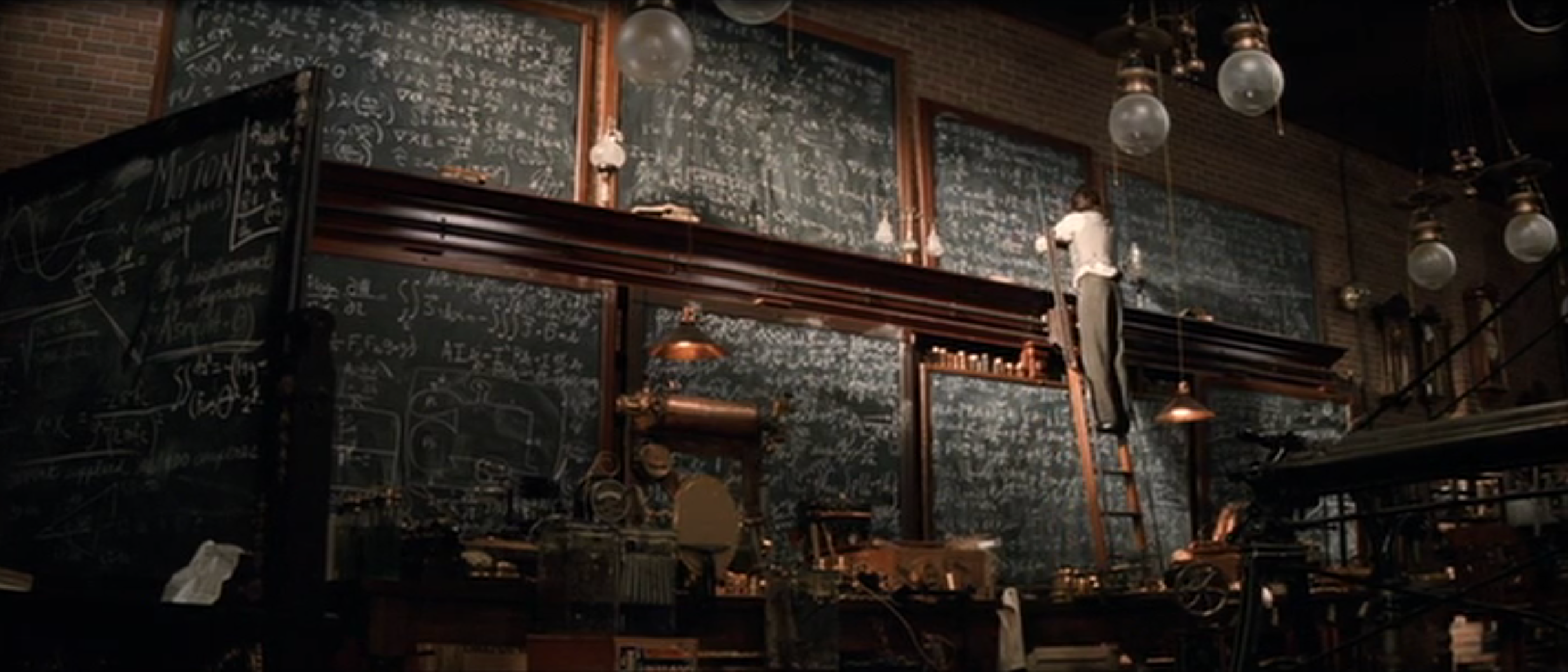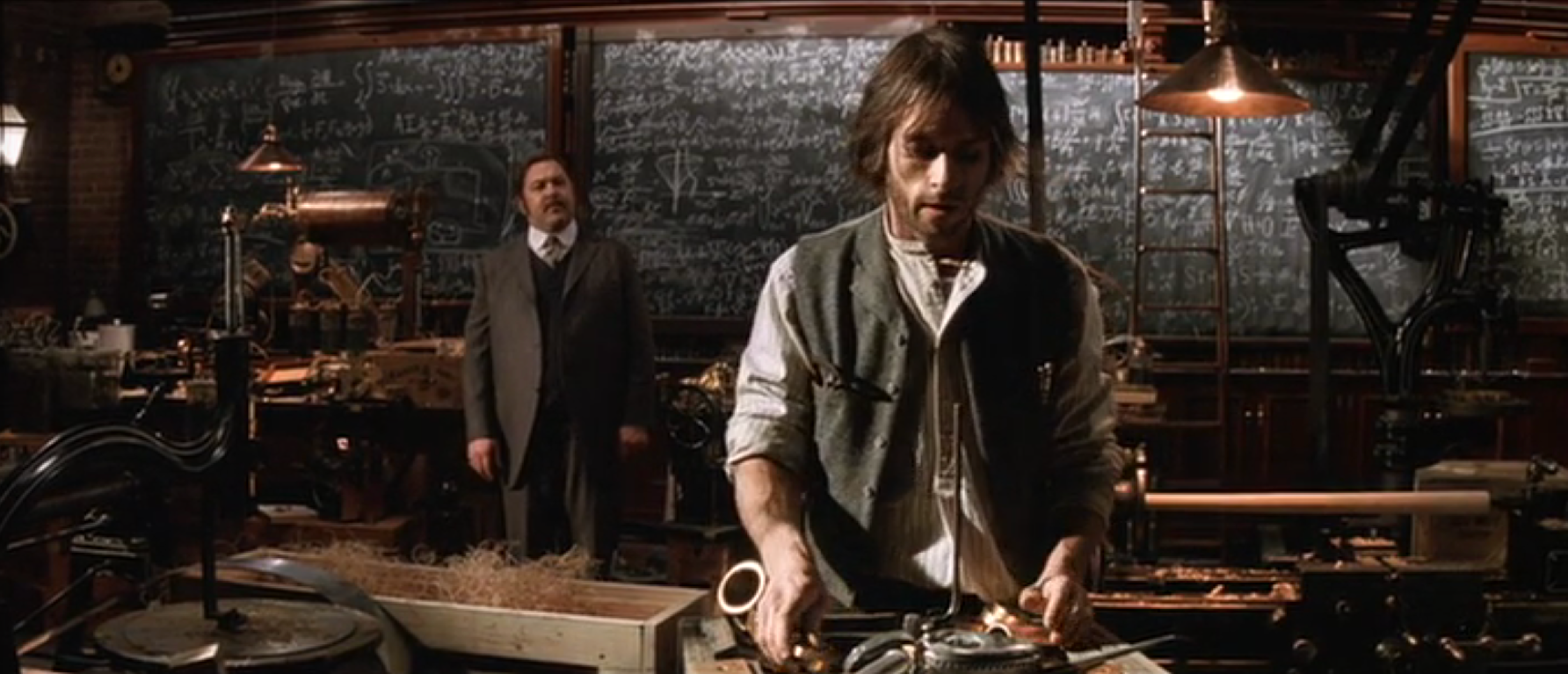Oliver Knill, Posted April 13, 2019,